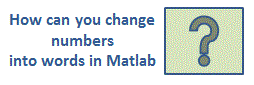# Check Writer – how to manipulate strings in Matlab

 This Matlab program is a ‘check writer’. You must provide a numerical date, payee of the check, and amount to be written (any number between 1.00 and 999,999.99 dollars). The program translates the date and amount to words, and prints providing some spacing within the check (easily modifiable). We achieve this task using simple string concatenation in Matlab.

You should regard the listing as a sample of a check-writing code. The algorithm for translating numbers into words (or working with strings) is general and may be easily adapted to other programming languages.

There are five sections to convert numbers into words in this Matlab program.

• Processing the thousands
• Processing the hundreds
• Processing the tens
• Processing cents
• Main code that accesses and controls the other four sections

We use cell arrays to handle the necessary strings, and the process consists in finding the correct index of an initial given ‘dictionary’.The Matlab function to work with the first three digits (thousands) is as follows. The string (named ‘words’) is updated after each digit is evaluated.

function [words, h, u] = thousands(amount)
global number

words = [];
% Find if there are thousands
t = floor(amount/1000);
if (t == 0)
h = 0;
u = 0;

return
end

% Find the hundreds
h = floor(amount/100000);

if h > 0
words = [number{h}
' HUNDRED'];
end

% Find the last two digits
u = floor(amount/1000) - h*100;
if (0 < u) & (u < 21)
words = [words
' ' number{u}];
else
words = [words tens(u, [])];
end

% End string with word 'thousand'
words = [words ' THOUSAND'];

The 'check writer' function to work with the three digits previous to the decimal point (hundreds) is as follows. Again, the string (named ‘words’) is updated after each digit is evaluated and the Matlab algorithm flows in a similar way than before.

function words = hundreds(amount, words, h, u)
global number

% Find if there are hundreds
hu = floor(amount - h*1e5 - u*1e3);
if (hu == 0)
words = [words];

return
end

% Find the hundreds
h = floor(hu/100);
if h > 0
words = [words
' ' number{h} ' HUNDRED'];
end

% Find the last two digits
u = hu - h*100;
if (0 < u) & (u < 21)
words = [words
' ' number{u}];
else
words = [words tens(u, [])];
end

The section to translate the last two digits of each group of three elements is given below. Note that the index to locate the correct number in the given dictionary (defined in the control section, below) works only for numbers beyond the first 20 elements in the list, due to the way that English syntax works.  For the first 20 elements, it 's not necessary to go through this script.

function words = tens(u, words)
global number

% Work with the last two digits of the group of three
if u == 0
words = [words];

return
end

% Find the correct index of the appropriate multiple of 10
words = [words ' ' number{floor((u - 20)/10 + 20)}];
units = u - floor(u/10)*10;

if units == 0

return
end

% Add a dash and the appropriate number if there are units
words = [words '-' number{units}];

Now, this is the function to add the remaining cents to the almost ready final string. It converts numbers into strings using command 'num2str'.

function words = cents(amount, words)
c = amount*100 - floor(amount)*100;

if c < 10
cents = [
'0' num2str(c)];
else
cents = num2str(c);
end

words = [words ' AND ' cents '/100'];

The control module that accesses the previous four routines for our check writer is as follows (name it check_writer.m, so you can run it from the command window):

clc; clear
global number

month = {
'January', 'February', 'March', 'April', 'May',...
'June', 'July', 'August','September', 'October',...
'November', 'December'};

number = {'ONE', 'TWO', 'THREE', 'FOUR', 'FIVE',...
'SIX', 'SEVEN', 'EIGHT', 'NINE', 'TEN',...
'ELEVEN', 'TWELVE', 'THIRTEEN', 'FOURTEEN', 'FIFTEEN',...
'SIXTEEN', 'SEVENTEEN', 'EIGHTEEN', 'NINETEEN',...
'TWENTY', 'THIRTY', 'FORTY', 'FIFTY',...
'SIXTY', 'SEVENTY', 'EIGHTY', 'NINETY'};

disp('Check Writer -----')
disp(
' ')

dat = input('Enter date (MMDDYY): ', 's');
m = str2num(dat(1:2));

name = input('Name of payee: ', 's');

a = input('Amount of check (include two decimals): ', 's');
amount = str2num(a);

if (amount < 1)|(amount > 999999.99)
disp(
'Sorry, amount is out of range...')

return
end

x = input('Prepare printer (enter when ready)... ', 's');
disp(
' ')

dat_str = [
'                                           '...
month{m} ' ' dat(3:4) ', ' '20' dat(5:6)];
disp(dat_str)

amount_str = ['                                           '...
'\$' a];
disp(amount_str)

name_str = ['                     ' name];
disp(name_str)

[words, h, u] = thousands(amount);
words = [hundreds(amount, words, h, u)];
words = [cents(amount, words)
' DOLLARS'];
disp(words)

Now, let’s try our code, let's change numbers into words for a good application:

Check Writer -----

Enter date (MMDDYY): 122309
Name of payee: Heavenly Bank of Algorithmland
Amount of check (include two decimals): 23999.00

December 23, 2009

\$23999.00

Heavenly Bank of Algorithmland

TWENTY-THREE THOUSAND NINE HUNDRED NINETY-NINE AND 00/100 DOLLARS

Another run:

Check Writer -----

Enter date (MMDDYY): 010110
Name of payee: Matrixlab-Examples.com
Amount of check (include two decimals): 734100.15

January 01, 2010

\$734100.15

Matrixlab-Examples.com

SEVEN HUNDRED THIRTY-FOUR THOUSAND ONE HUNDRED AND 15/100 DOLLARS

From 'Check Writer' to home

From 'Check Writer' to 'Matlab Programming'